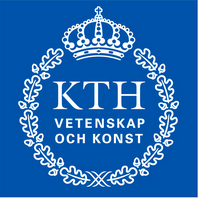## Fast Iterative Solution of the Time-harmonic Elastic Wave Equation at Multiple Frequencies

##### SHELL Monitor: Dr. René-Édouard Plessix

Fast and accurate simulation of wave propagation through an elastic medium is a key component in geophysical exploration. By assuming a time-harmonic wave field and after discretizing the governing equation, the wave can be computed by solving a large complex and indefinite system of linear equations. This time-harmonic elastic wave equation has the same structure as the Helmholtz equation for acoustic wave propagation in the sense that both can be written as $(K + if C -f^2 M)\mathbf{x} = \mathbf{b}$. Here $f$ is the (angular) frequency, $i = \sqrt{-1}$ is the imaginary unit number, $K$, $C$ and $M$ are symmetric and positive definite matrices and the right-hand side vector $\mathbf{b}$ corresponds to a source term. Such systems typically have to be solved at several frequencies and for dozens of right-hand side vectors $\mathbf{b}$. The grid size must be small enough to describe a wave, which results in very large systems for high frequencies (many millions of linear equations) that are hard to solve due to the indefiniteness of the system matrix. The time-harmonic elastic wave equation is even more difficult to solve than the Helmholtz equation since the number of unknowns per grid points is three instead of one, resulting in an even larger system, with a system matrix that is more dense (more non-zeros per row) and more ill-conditioned. My project aims to develop an efficient iterative mehtod for solving the time-harmonic elastic wave equation at multiple frequencies and for multiple source terms. In order to develop this method, algorithms that have been successfully applied for the Helmholtz equation will be generalized for the time-harmonic elastic wave equation. We aim for (nearly) frequency and grid independent convergence so that the solver can be successfully applied to problems at the relevant frequencies for geophysical applications.

## Studies and early projects (2008-2013)#### BSc Theses• M. Baumann and P. Buran (2011). Numerical Solution of the Vorticity Transport Equation on Nvidia GPUs. BSc Thesis, Technical University of Berlin. (abstract, report, slides)
• Scientific supervision: Günter Bärwolff

#### Others• Project work on Balanced Truncation with M. Ambrozic and C. Echeverria (report, slides)

• A poster on SPH I did with A. Vajaykumar and S. Milovanovic. Please also see the pictures of the poster presentation (Pic1, Pic2)